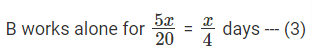# CAT Prep 2022 | CAT Previous Year Question Papers With Solution | CAT 2022 Question Bank | PaGaLGuY

CAT 2019 Quant Question: Profit & loss

A shopkeeper sells two tables, each procured at cost price p, to Amal and Asim at a profit of 20% and at a loss of 20%, respectively. Amal sells his table to Bimal at a profit of 30%, while Asim sells his table to Barun at a loss of 30%. If the amounts paid by Bimal and Barun are x and y, respectively, then (x - y) / p equals

A. 1
B. 1.2
C. 0.7
D. 0.50

The Shopkeeper procures the table at price ‘p’

He gains 20% on the transaction with Amal
So, Amal buys the table at ‘1.2p’
Amal sells athe table at 30% profit,
So the Selling Price of Amal = 1.3 * 1.2p = 1.56p
x = 1.56p

The Shopkeeper looses 20% on the transaction with Asim
So, Asim buys the table at ‘0.8p’
Asim sells athe table at 30% loss,
So the Selling Price of Asim = 0.7 * 0.8p = 0.56p
y = 0.56p

(x-y)/p = (1.56p - 0.56p)/p = 1.

Hence, the answer is 1

Choice A is the correct answer.

CAT 2019 Quant Question: Work & time

John gets Rs. 57 per hour of regular work and Rs. 114 per hour of overtime work. He works altogether 172 hours and his income from overtime hours is 15% of his income from regular hours. Then, for how many hours did he work overtime? [TITA]

If John works the same number of regular and over-time hours say ‘p’
The the income would be 57p and 114p

Let’s say that he works ‘x’ hours regular and ‘y’ hours overtime…
So, the income would be 57x and 114y

we are told that 114y is 15% of 57x
114y = 0.15 * 57x
y = 0.075x

we also know that x+y = 172
therefore, x + 0.075x = 1.075x = 172
x = 160

y = 172 - 160 = 12
Therefore, the number of hours he worked Overtime is 12.

Hence, the answer is 12

CAT 2018 Quant Question: Mixtures and Alligations

A trader sells 10 litres of a mixture of paints A and B, where the amount of B in the mixture does not exceed that of A. The cost of paint A per litre is Rs. 8 more than that of paint B. If the trader sells the entire mixture for Rs. 264 and makes a profit of 10%, then the highest possible cost of paint B, in Rs. per litre, is

A. 20
B. 16
C. 22
D. 26

Given, The amount of B in mixture ≤ Amount of A in mixture
Selling Price = Rs. 264 and Profit = 10 %
264 = 1.1 × CP
CP =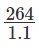= Rs. 240 for 10 litres
CP per litre = Rs. 24
We also know that, CP per litre of A = CP per litre of B + 8 Let CP per litre of B = x So, CP per litre of A = x + 8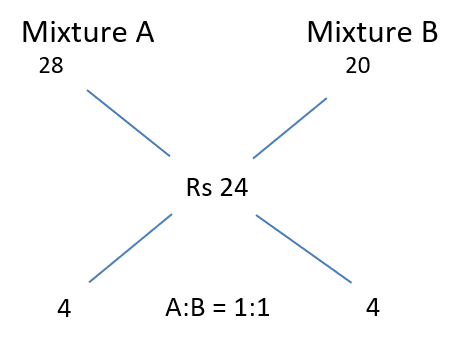Given Amount of B ≤ Amount of A,
Maximum possible cost of B occurs when B = A
We need to choose values for A and B in such a way that the quantities remain same.But A should be 8 more than B
Therefore, A should have a CP per litre of Rs. 28 and B should have a CP per litre of Rs 20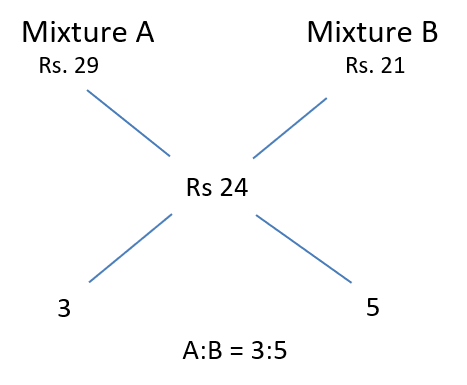B cannot be assigned any more than this as the amount of B would become more than A
Example: Let us assign B to be Rs. 21 and A to be Rs. 29 which would result in a ratio of 3:5 where B amounts more than A, which doesn’t satisfy the condition
Max possible cost of Paint B = Rs. 20 per litre

Hence, the answer is Rs.20 per litre

Choice A is the correct answer.

CAT 2018 Quant Question: Geometry

In a circle with centre O and radius 1 cm, an arc AB makes an angle 60 degrees at O. Let R be the region bounded by the radii OA, OB and the arc AB. If C and D are two points on OA and OB, respectively, such that OC = OD and the area of triangle OCD is half that of R, then the length of OC, in cm, is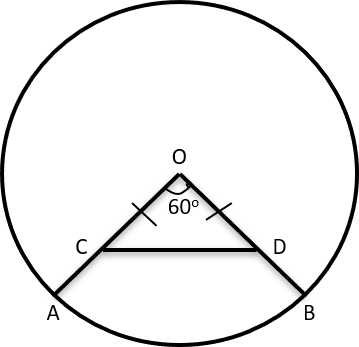Given ∠AOB = 60°
Area of Sector AOB =Given OC = OD => ∠OCD = ∠ODC = 60°
△OCD is an Equilateral Triangle with side = aHence, the answer is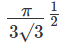cm

Choice D is the correct answer.

CAT 2018 Quant Question: Functions

If f(x + 2) = f(x) + f(x + 1) for all positive integers x, and f(11) = 91, f(15) = 617, then f(10) equals. [TITA]

Given, f(x+2) = f(x) + f(x+1) f(11) = 91, f(15) = 617
We get 91 + f(12) = f(13)

Let f(12) be equal to some value ‘a’
So, 91 + a = f(13).

f(12) + f(13) = f(14)
a + 91 + a = f(14)
So, f(14) = 2a + 91 f(13) + f(14) = 617
So, 91 + a + 2a + 91 = 617
3a + 182 = 617
a = 145

Substituting the value of a and f(11), we get
f(10)+ 91 = 145
f(10) = 54

Hence, the answer is 54.

CAT 2018 Quant Question: Speed Time Distance

The distance from A to B is 60 km. Partha and Narayan start from A at the same time and move towards B. Partha takes four hours more than Narayan to reach B. Moreover, Partha reaches the mid-point of A and B two hours before Narayan reaches B. The speed of Partha, in km per hour, is

A. 6
B. 3
C. 4
D. 5Let Narayanan take X hrs to reach B then Partha would take X + 4 hrs
Its given that Partha reaches the mid-point of A and B two hours before Narayan reaches B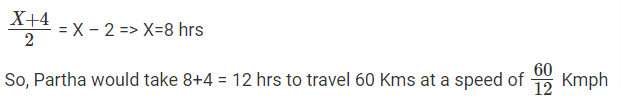Speed of Partha = 5 Kmph

Hence, the answer is 5 Kmph

Choice D is the correct answer.

CAT 2018 Quant Question: Averages

A CAT aspirant appears for a certain number of tests. His average score increases by 1 if the first 10 tests are not considered, and decreases by 1 if the last 10 tests are not considered. If his average scores for the first 10 and the last 10 tests are 20 and 30, respectively, then the total number of tests taken by him is [TITA]

Let there be ‘n’ tests and the Overall Average score be k
Average (n) = k => Total marks = nk
So, when we ignore the first 10 questions,
Average (n-10) = k+1
Similarly, if we ignore the last 10 questions,
Average (first (n-10)) = k-1

It is given that when the first 10 tests are not considered, the overall average increases by 1 (Each question carries 20 marks)
Total marks – 20×10 = (k+1) (n-10)
kn – 200 = (k+1) (n-10) —(1)
Similarly, If the last 10 tests are not considered, the overall average decreases by 1 (Each question carries 30 marks)
kn - 300 = (k-1) (n-10) —(2)

Solving (1) and (2), we get
kn - 200 = (k+1) (n-10)
(-) kn - 300 = (k-1) (n-10)

100 = 2(n-10)
50 = n-10
n = 60 questions

Hence, the answer is 60 questions

CAT 2018 Quant Question: Ratio & Proportions

Two types of tea, A and B, are mixed and then sold at Rs. 40 per kg. The profit is 10% if A and B are mixed in the ratio 3 : 2, and 5% if this ratio is 2 : 3. The cost prices, per kg, of A and B are in the ratio

A. 21 : 25
B. 19 : 24
C. 18 : 25
D. 17 : 25

Let Tea A and B be sold at Rs. a and Rs. b respectively
When A and B are mixed in the ratio 3 : 2 and sold at a profit of 10% at Rs.40 per KgWhen A and B are mixed in the ratio 2 : 3 and sold at a profit of 5% at Rs.40 per Kg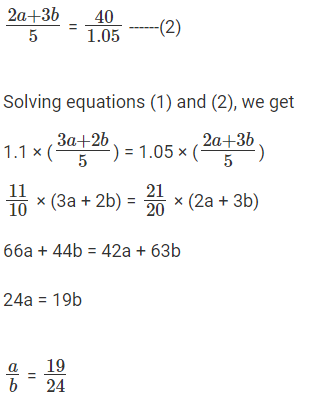So, the cost prices per Kg of A and B are in the ratio 19 : 24

Hence, the answer is 19 : 24

Choice B is the correct answer.

CAT 2018 Quant Question: Mixtures

A wholesaler bought walnuts and peanuts, the price of walnut per kg being thrice that of peanut per kg. He then sold 8 kg of peanuts at a profit of 10% and 16 kg of walnuts at a profit of 20% to a shopkeeper. However, the shopkeeper lost 5 kg of walnuts and 3 kg of peanuts in transit. He then mixed the remaining nuts and sold the mixture at Rs. 166 per kg, thus making an overall profit of 25%. At what price, in Rs. per kg, did the wholesaler buy the walnuts?

A. 84
B. 86
C. 96
D. 98

Let the wholesaler bought walnuts and peanuts at Rs 3x and Rs x respectively
He sold 8kgs of Peanuts to the shopkeeper at 10% Profit
Cost price of the Peanuts bought by the shopkeeper = Rs. 1.1x per kg
Similarly, he sold 16 kgs of Walnuts to the shopkeeper at 20% Profit
Cost price of the Walnuts bought by the shopkeeper = 1.2 × 3x = Rs. 3.6x per Kg

He lost 5 kgs of Walnuts and 3 Kgs of Peanuts in transit
Remaining = 16 - 5 = 11 kgs of Walnuts & 8-3 = 5 kgs of Peanuts
He mixes them together and sells them at Rs. 166 per kg, making an overall Profit of 25%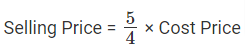Overall Cost Price = Rs.(3.6 × 16x + 1.1 × 8x)
Overall Selling Price = Rs. 16 × 166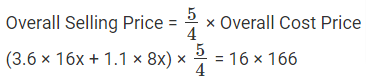( ( 57.6 × 8.8) x ) × 5/4 = 16 × 166
x = Rs. 32
Cost price per Kg of Walnuts bought by the Wholesaler = Rs. 3x = 3 × 32 = >Rs. 96

Hence, the answer is Rs.96

Choice C is the correct answer.

CAT 2018 Quant Question: Work and Time

When they work alone, B needs 25% more time to ﬁnish a job than A does. They two ﬁnish the job in 13 days in the following manner: A works alone till half the job is done, then A and B work together for four days, and ﬁnally B works alone to complete the remaining 5% of the job. In how many days can B alone ﬁnish the entire job?

A. 16
B. 22
C. 20
D. 18